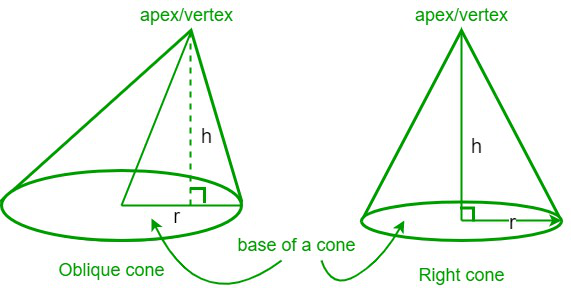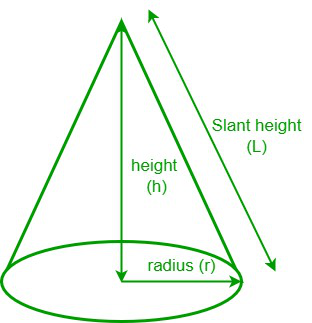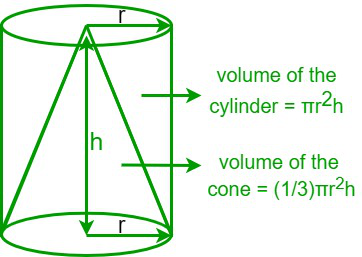Open in App
Not now

# How to find the Volume of a Cone?

• Last Updated : 23 May, 2022

In geometry, a cone is a three-dimensional figure with a flat surface, and a curved surface pointed toward the top, where the pointed end is called the apex or a vertex. A right circular cone is a cone having its vertex above the center of the cone, and it is generated by rotating a right-angled triangle about one of its legs. On the other hand, an oblique cone doesn’t have its vertex above the center of the cone, i.e., the axis of the cone is not perpendicular to its base. The height of a cone is the distance from the center of the base to the vertex of a cone, whereas the slant height is the distance from the vertex of a cone to any point on the circumference of its circular base. Here are some examples of a cone that we see in our daily life: an ice-cream cone, a funnel, birthday hats, etc.### Volume of a cone

The volume of a cone is defined as the amount of space enclosed by a cone, and it is usually measured in cubic meters, cubic centimeters, cubic inches, etc. The formula for the volume of a cone is equal to one-third of the product of the area of the base and the height of the cone. It is generally represented by the letter “V”.Formula for the volume of a cone is given as follows,

Volume of a cone (V) = (1/3) π r2 h cubic units

Where r is the radius of the circular base,

And h is the height of the cone

### Volume of the cone in terms of slant height

The slant height is defined as the distance from the vertex of a cone to any point on the circumference of its circular base. From the above figure, we can notice that a right-angled triangle is formed between the base radius, height, and slant height. So, by applying the Pythagorean theorem, we get

L2 = r2 + h2

r = √(L2 -h2)

h = √(L2 – r2)

• Volume of the cone in terms of the slant height (L) and the height (h) is,

Volume of a cone (V) = (1/3) π(L2 – h2)h

• Volume of the cone in terms of the slant height (L) and the base radius (r) is,

Volume of a cone (V) = (1/3) πr2√(L2 – r2)

Derivation of the volume of a cone

Let’s consider that a conical flask is filled with oil. Now, to calculate the amount of oil filled in the conical flask, we need to know the capacity of the conical flask. We know that the capacity of the conical flask is generally equal to the volume of the cone. Now take a cylindrical tin that has the same base radius and the same height as the given conical flask. Now transfer the oil present in the conical flask to the cylindrical tin. We could notice that the cylindrical tin was not completely filled. So, repeat the experiment once more, and we can notice that there is still some vacant space in the cylindrical tin. Once again, repeat the experiment, and this time we can notice that the tin is filled up to the brim. Hence, from the observation, we can conclude that the volume of a cylinder is equal to three times the volume of a cone that has the same base radius (r) and the same height (h) as the cylinder.So,

Volume of a cylinder = 3 × [The volume of a cone]

i.e.,

Volume of a cone = (1/3) × [The volume of a cylinder]

We know that,

Volume of a cylinder = πr2h cubic units

Hence,

Volume of a cone (V) = (1/3) πr2h cubic units

Where r is the base radius and h is the height of the cone.

Determining the volume of a cone

Let’s consider an example to determine the volume of a cone.

Example: Determine the volume of a cone if the radius of its circular base is 3 cm and the height is 5 cm.

Step 1: Note the radius of the circular base (r) and the height of the cone (h). Here, the radius is 3 cm and the height is 5 cm.

Step 2: Calculate the area of the circular base = πr2. Substitute the value of r and π in the given equation, i.e., 3.14 × (3)2 = 28.26 cm2.

Step 3: We know that the volume of a cone is (1/3) × (area of the circular base) × height of the cone. So, substitute the values in the equation = (1/3) × 28.26 × 5 = 47.1 cm3.

Step 4: Hence, the volume of the given cone is 47.1 cm3.

### Sample Problems

Problem 1: Calculate the volume of a cone if the radius of the circle is 4 cm and the height of the cone is 6 cm.

Solution:

Given data,

Height (h) = 6 cm

We know that,

Volume of a cone (V) = (1/3) πr2h cubic units

⇒ V = (1/3) × 3.14 × (4)2 × 6

⇒ V = (1/3) × 3.14 × 16 × 6 = 100.48 cm3

Hence, the volume of the given cone is 100.48 cm3.

Problem 2: Find the height of the cone if its volume is 210 cm3 and the radius of the circular base is 3.5 cm. [Take π = 22/7]

Solution:

Given data,

Volume of the cone = 210 cm3

Radius of the circular base = 3.5 cm.

We know that,

Volume of a cone (V) = (1/3) πr2h cubic units

⇒ 210 = (1/3) × (22/7) × (3.5)2 × h

⇒ h = 16.36 cm

Hence, the height of the cone is 16.36 cm.

Problem 3: Find the volume of the cone if the diameter of the circular base is 10 cm and the height is 8 cm.

Solution:

Given data,

Height (h) = 8 cm

Diameter (d) = 2r = 10 cm

⇒ Radius (r) = 5 cm

We know that,

Volume of a cone (V) = (1/3) πr2h cubic units

⇒ V = (1/3) × (3.14) × (5)2 × 8

⇒ V = (1/3) × 3.14 × 25 × 8 = 209.34 cm3

Hence, the volume of the cone = 209.34 cm3

Problem 4: Calculate the diameter of the circular base of a cone if its volume is 264 cubic inches and its height is 7 inches. [Take π = 22/7]

Solution:

Given data,

Volume of the cone = 264 cubic inches

Height of the cone = 7 inches

We know that,

Volume of a cone (V) = (1/3) πr2h cubic units

⇒ 264 = (1/3) × (22/7) × r2 × (7)

⇒ r2 = 36 ⇒ r = √36 = 6 inches

Diameter = 2r = 2 × 6 = 12 inches

Hence, the diameter of the circular base is 12 inches.

Problem 5: Determine the volume of a cone if the radius of its circular base is 5 units and the slant height is 13 units.

Solution:

Given data,

Slant height (L) = 13 units.

We know that,

Slant height (L) = √(r2 + h2)

⇒ height (h) = √(L2 – r2) = √[(13)2 – (5)2]

⇒ h = √(169 – 25) = √144 = 12 units

We have,

Volume of a cone (V) = (1/3) πr2h cubic units

⇒ V = (1/3) × (3.14) × (5)2 × (12)

⇒ V = (1/3) × (3.14) × (25) × (12) = 314 cubic units

Hence, the volume of the given cone is 314 cubic units.

Problem 6: Find the volume of a cone if its slant height is 10 inches and height is 8 inches.

Solution:

Given data,

Height of cone (h) = 8 inches

Slant height (L) = 10 inches

We know that,

Slant height (L) = √(r2 + h2)

⇒ radius (r) = √(L2 – h2) = √[(10)2 – (8)2]

⇒ r = √(100 – 64) = √36 = 6 inches

We have,

Volume of a cone (V) = (1/3) πr2h cubic units

⇒ V = (1/3) × (3.14) × (6)2 × (8)

⇒ V = (1/3) × (3.14) × 36 × 8 = 301.44 cubic inches

Hence, the volume of the given cone is 301.44 cubic inches.

My Personal Notes arrow_drop_up
Related Articles